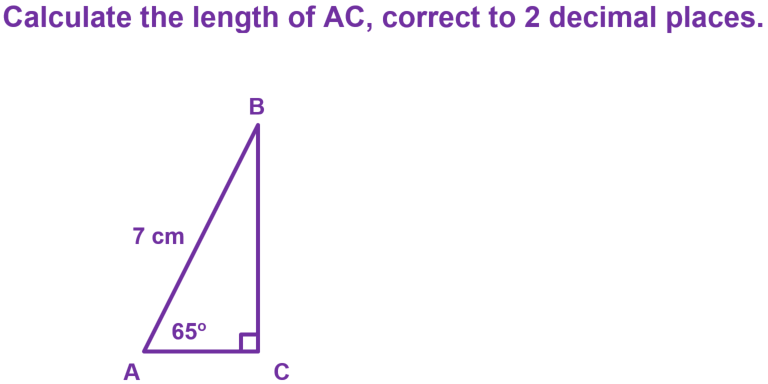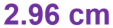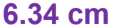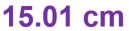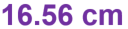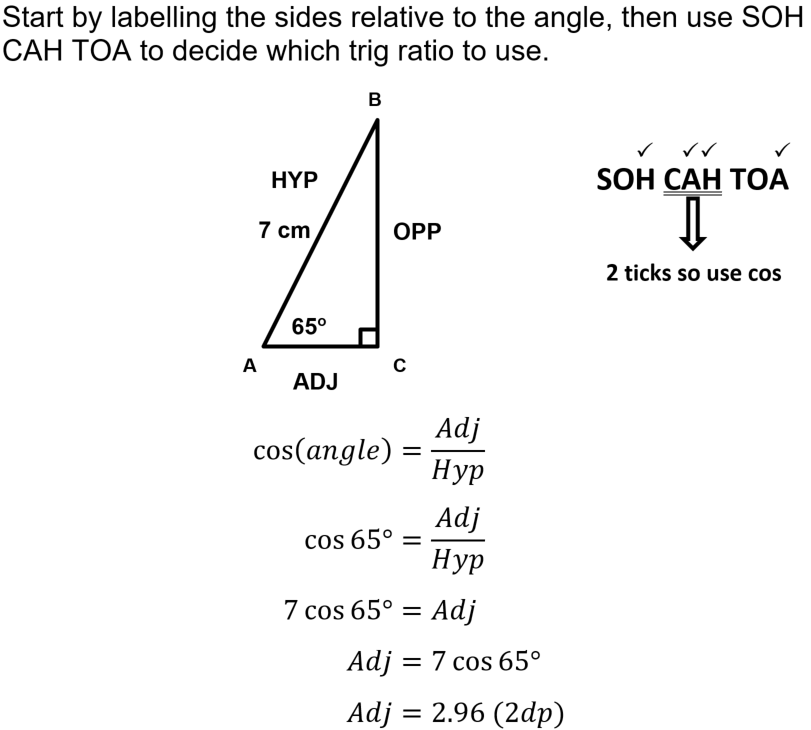See if you can get full marks in this maths quiz.

# Right-Angled Trigonometry (F)

Trigonometry is the final jigsaw piece that brings together everything that matters to do with right-angled triangles. Pythagoras' Theorem works when we know two sides and need to find the third, and our basic angle facts allow us to work out the missing angle if we know two of them. Trigonometry is used when we have an angle and a side – from this, we can work out all the missing lengths. This GCSE Maths quiz will test you on sine, cosine and tangent.

Right-angled trigonometry is usually remembered by the mnemonic SOH CAH TOA. In order to use it correctly, you also need to label the sides as Opposite or Adjacent to the angle given, and don’t forget to label the Hypotenuse too. Tick off in SOH CAH TOA what you need to find out, the one with two ticks is the ratio to use.

The real beauty of trigonometry is that it doesn’t just apply to right-angled triangles. The Sine and Cosine rules can be applied to triangles of any shape, and combining a bit of trigonometry with the formula for the area of a triangle comes up with a new rule, which works when you know two sides and the included angle.

Trigonometry goes well beyond 90o, and well beyond triangles. Waves, be they sound waves or sea waves, can be modelled using trigonometry, and it comes into the equations of motion of objects that are thrown both forward and upward, like a cricket ball or javelin. It comes right into your home, as without trigonometry there would be no AC power distribution!

Question 1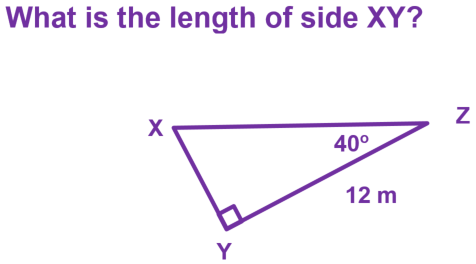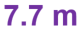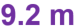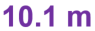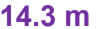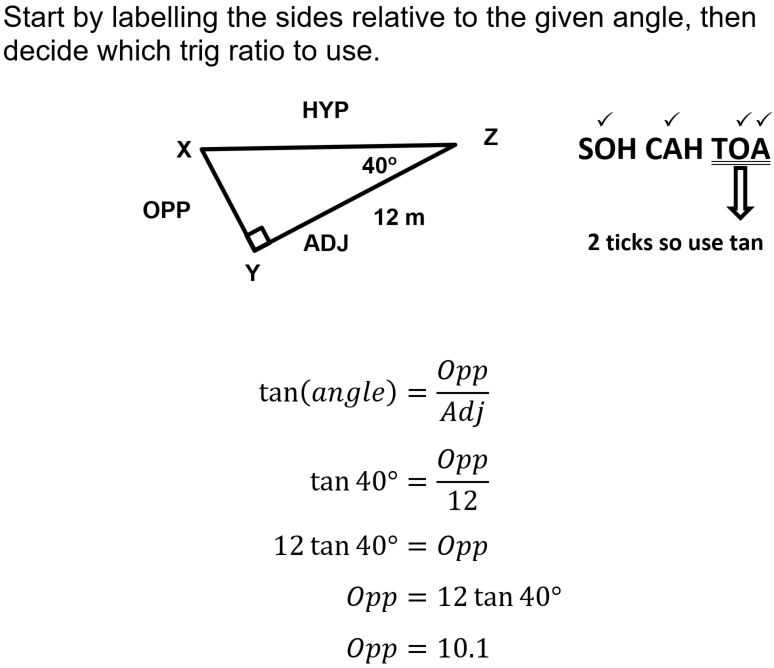Question 2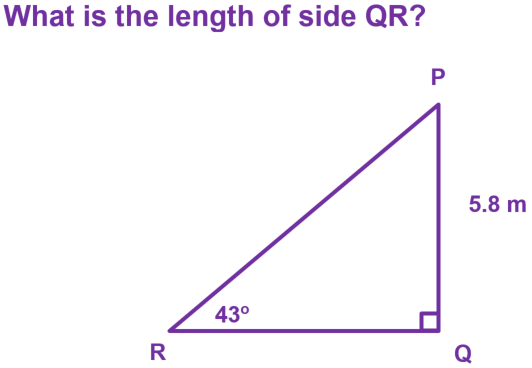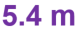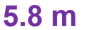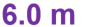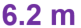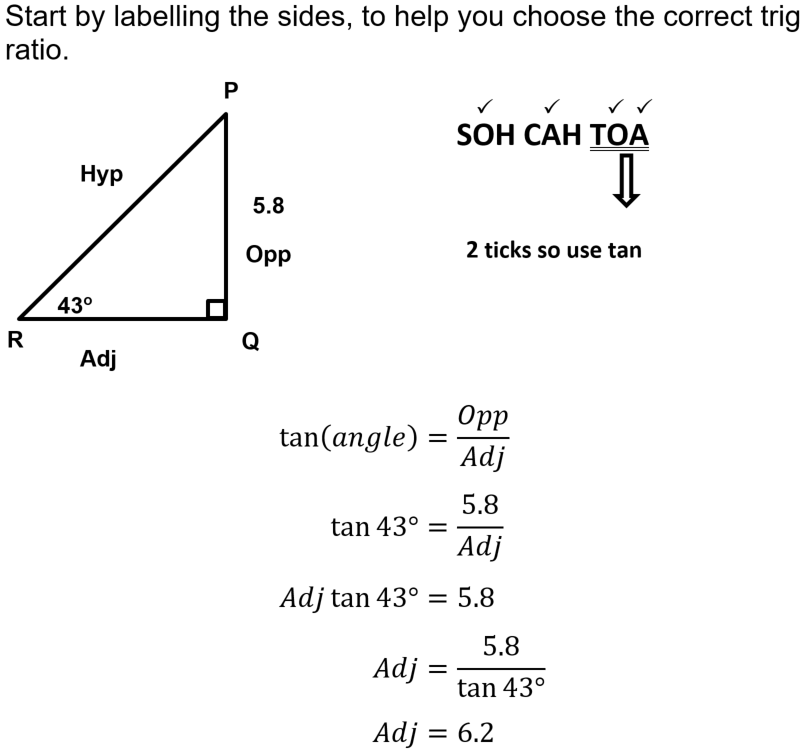Question 3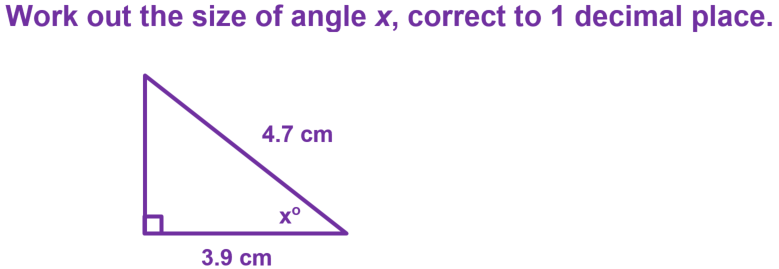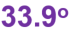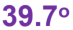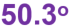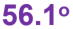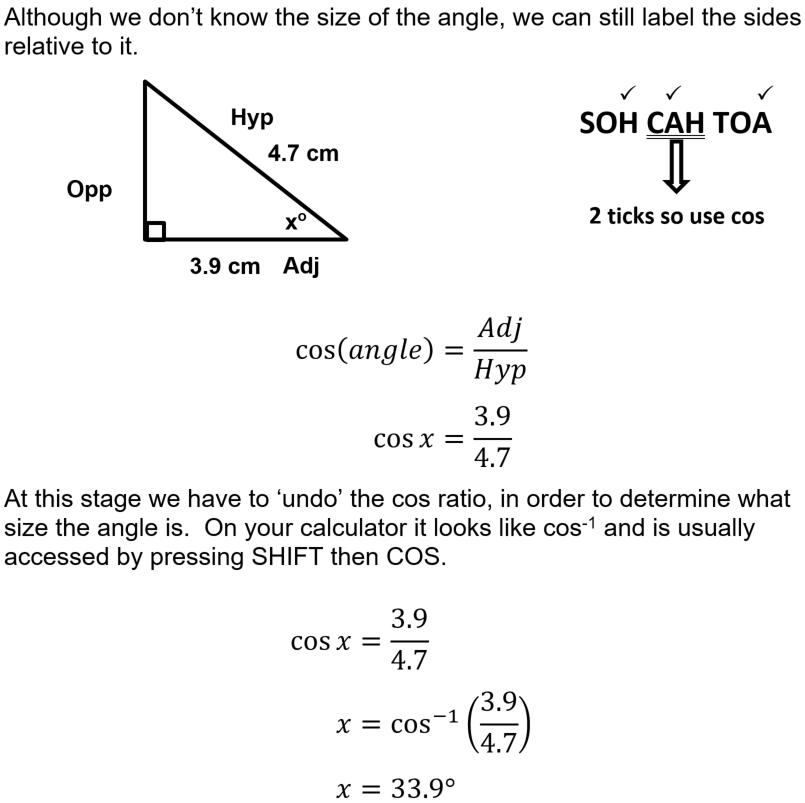Question 4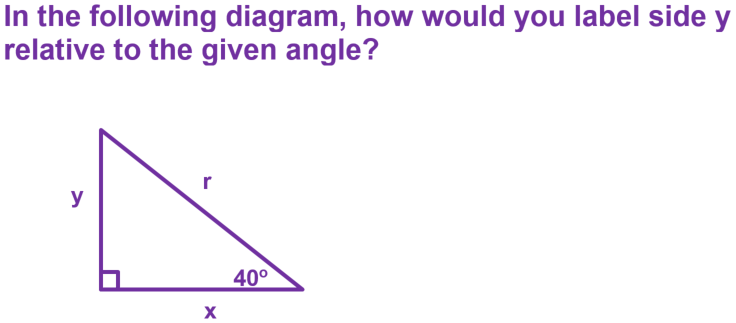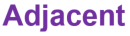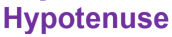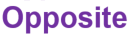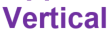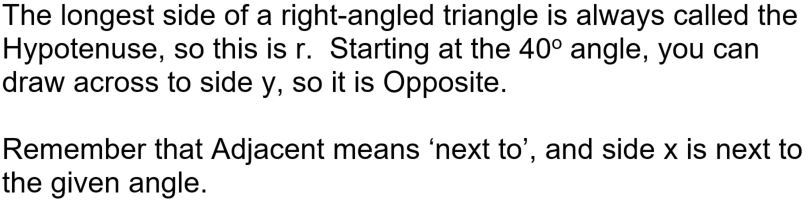Question 5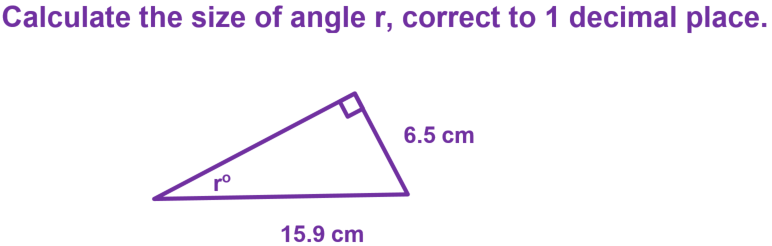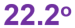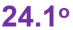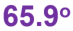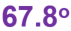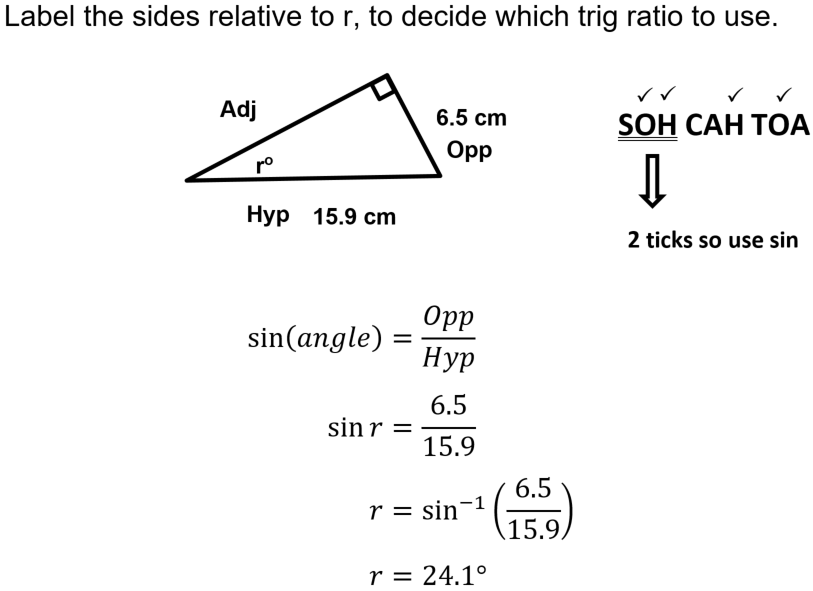Question 6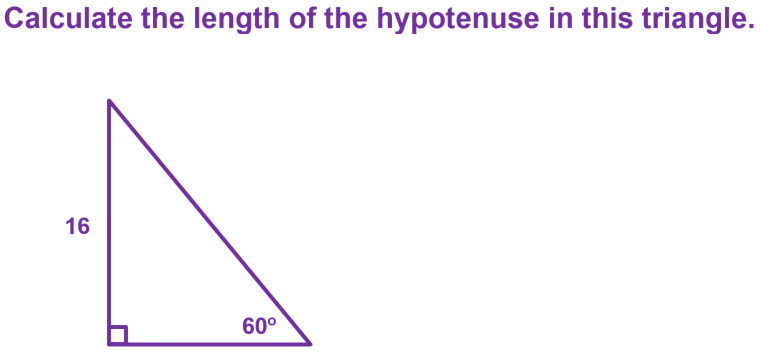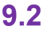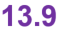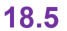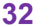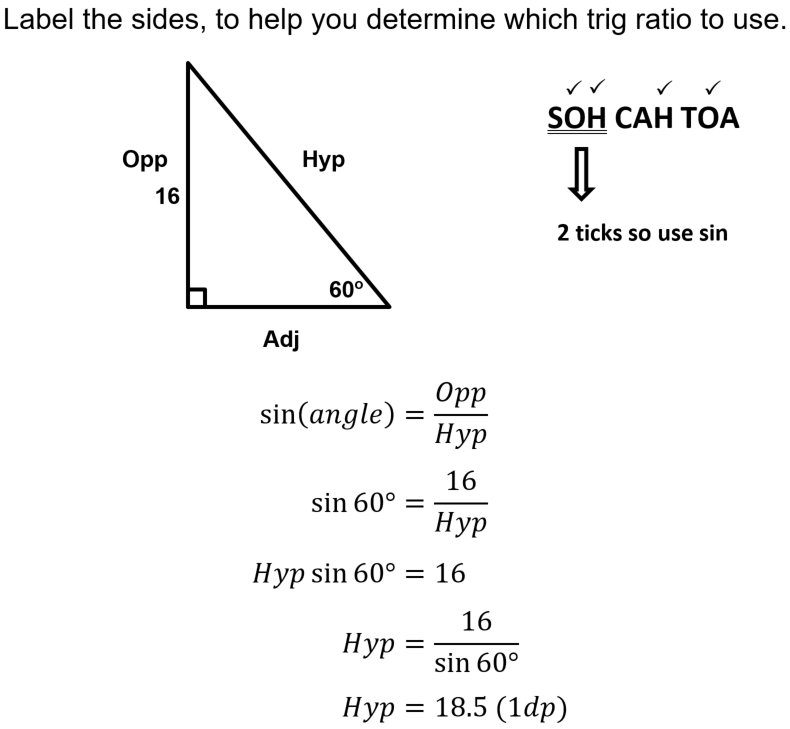Question 7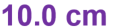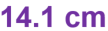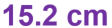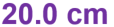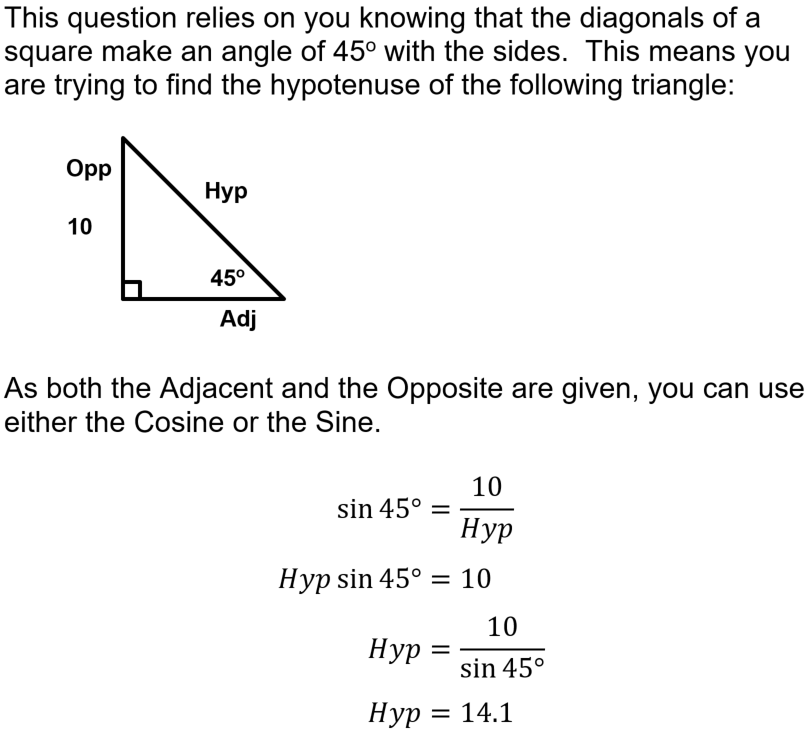Question 8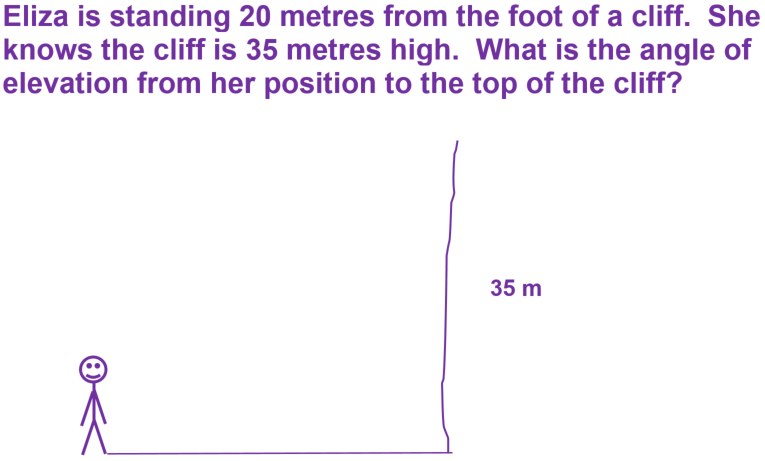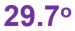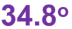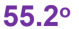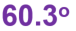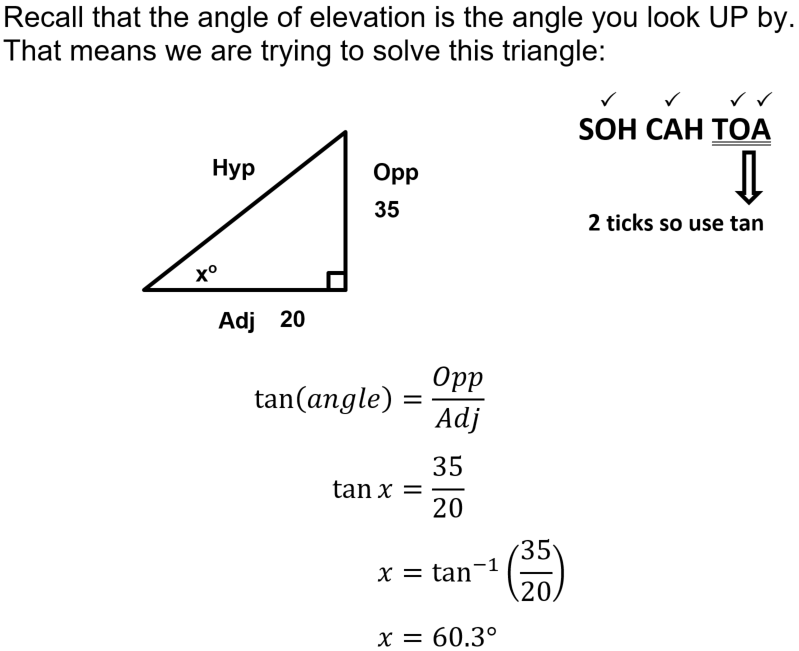Question 9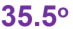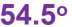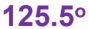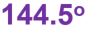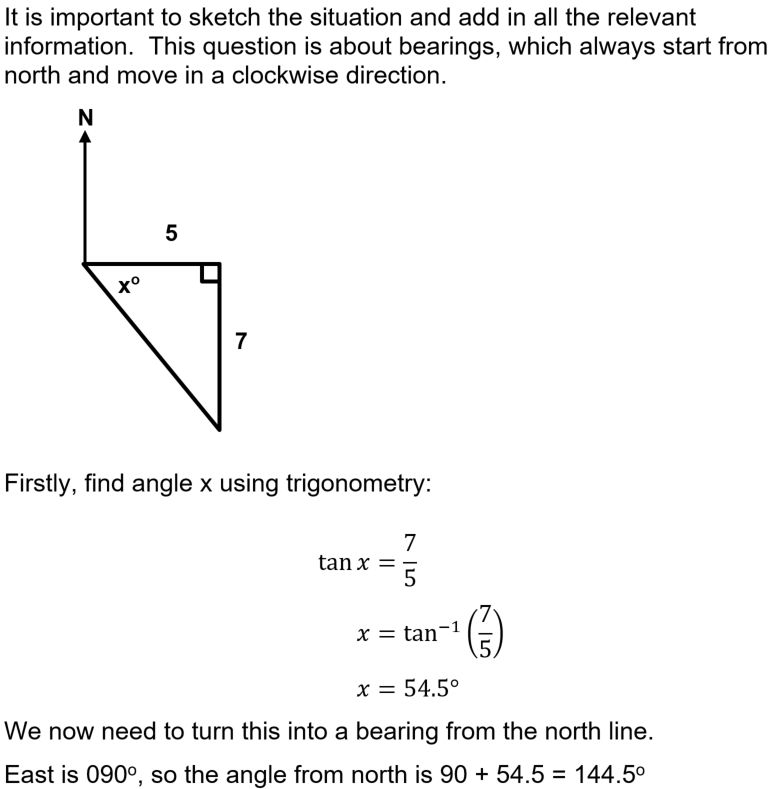Question 10